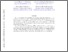# Constant Inapproximability for PPA

Deligkas, Argyrios, Fearnley, John, Hollender, Alexandros and Melissourgos, Themistoklis
(2022) Constant Inapproximability for PPA. [Preprint]Text 2201.10011v1.pdf - Submitted Version Download (797kB) | Preview

## Abstract

In the $\varepsilon$-Consensus-Halving problem, we are given $n$ probability measures $v_1, \dots, v_n$ on the interval $R = [0,1]$, and the goal is to partition $R$ into two parts $R^+$ and $R^-$ using at most $n$ cuts, so that $|v_i(R^+) - v_i(R^-)| \leq \varepsilon$ for all $i$. This fundamental fair division problem was the first natural problem shown to be complete for the class PPA, and all subsequent PPA-completeness results for other natural problems have been obtained by reducing from it. We show that $\varepsilon$-Consensus-Halving is PPA-complete even when the parameter $\varepsilon$ is a constant. In fact, we prove that this holds for any constant $\varepsilon < 1/5$. As a result, we obtain constant inapproximability results for all known natural PPA-complete problems, including Necklace-Splitting, the Discrete-Ham-Sandwich problem, two variants of the pizza sharing problem, and for finding fair independent sets in cycles and paths.

Item Type: Preprint cs.CC, cs.CC, cs.GT Faculty of Science and Engineering > School of Electrical Engineering, Electronics and Computer Science Symplectic Admin 03 Feb 2022 13:22 26 May 2022 02:10 Author https://livrepository.liverpool.ac.uk/id/eprint/3148114